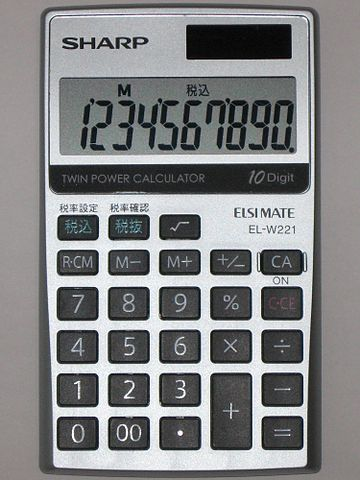# Eight EdditionsA malfunctioned calculator has only two keys working '8' and '+'. A calculation of 24 can be done by using the keys "8+8+8". What is the least number of times that the '8' key can be used to calculate the sum of 1000?

×